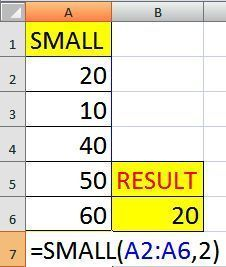### Latest Post

4/recent/ticker-posts

# Excel Static Function, Average, Averageif, Averageifs

Today we discuss in this artical use of important excel statistical functions AVERAGE, AVERAGEA, AVERAGEIF, AVERAGIFS, MAX, MIN, LARGE, SMALL & MEDIAN, so please read carefully.

## AVERAGE

This formula returns the average of selected range.

Syntax

AVERAGE(number1,number2,...)

Example - In this example given employee chart like employee id, employee name & employee salary. Here I want average of salary so lets start function =AVERAGE(C3:C8) then press enter and result display average of salary is 85500.

## AVERAGEA

This formula returns the average of selected range, including numbers, text, and logical values.

Syntax

AVERAGEA(number1,number2,...)

Example - In this example given employee chart like employee id, employee name & employee salary. Here I want average of salary including text so lets start function =AVERAGEA(C3:C8) then press enter and result display average of salary is 4000.

## AVERAGEIF

This formula returns the average of all the selected cells in a range that meet a given criteria

## Syntax

AVERAGEIF(range,criteria,average_range)

Example - In this example given employee chart like employee id, employee name & employee salary. Here I want average of salary with condition, employee "A" salary average so lets start function =AVERAGEIF(B3:B8,"A",C3:C8) then press enter and result display employee "A" average of salary is 666.67.

## AVERAGEIFS

This formula returns the average of all selected cells that meet multiple criteria.

## Syntax

AVERAGEIFS(average_range,criteria_range1,criteria1,criteria_range2, criteria2…)

Example - In this example I want average of salary employee "B" and Department "FINANCE" only so lets start function =AVERAGEIFS(C4:C9,B4:B9,"FINANCE",A4:A9,"B") then press enter and result display employee "B" and Department "FINANCE" average of salary is 400.

Microsoft Excel Easy Notes for Beginners

## MAX

This formula returns the maximum value in a list of selected cells.

Syntax
MAX(number1,number2,...)

Example- In this data we find Max number so start formula -
=MAX(A2:A6) and press enter result display Max no. is 60.

## MIN

This function returns the minimum value in a list of selected range.

## Syntax

MIN(number1,number2,...)

Example- In this data we find Min number so start formula -
=MIN(A2:A6) and press enter result display Min no. is 20.

## LARGE

This formula returns the k-th largest value in a data set

## Syntax

LARGE(array,k)

Example- In this data we find Large number 1 and 2, so start formula - =LARGE(A2:A6,1) and LARGE (A2:A6,2) and press enter result display Large No.1 is 60 and Large No.2 is 50.

## SMALL

This formula returns the k-th Smallest value in a data set.

## Syntax

SMALL(array,k)Example- In this data we find Small number 1 and 2, so start formula - =SMALL(A2:A6,1) and SMALL (A2:A6,2) and press enter result display Small No.1 is 10 and Small No.2 is 20.

## MEDIAN

This functions returns the median of the given numbers.

## Syntax

MEDIAN(number1,number2,...)

Example- In this data we find Median number, then start formula - =MEDIAN(A2:A6)  press enter result display MEDIAN No.is 40

## RANK

This formula returns the rank of a number in a list of numbers.

## Syntax

RANK(number,ref,order)

Example- In this data we find Rank of 40, then start formula - =RANK(40,A2:A6,1) here 1 is ascending order, press enter result display Rank of 40 is 3.

## Conclusion

I hope you better understand formulas AVERAGE, AVERAGEA, AVERAGEIF, AVERAGIFS, MAX, MIN, LARGE, SMALL & MEDIAN. If any questions and comments so please ask me. We try reply soon.